# JP6367332B2 - Inverter control device and motor drive system - Google Patents

## Info

Publication number
JP6367332B2
JP6367332B2 JP2016535264A JP2016535264A JP6367332B2 JP 6367332 B2 JP6367332 B2 JP 6367332B2 JP 2016535264 A JP2016535264 A JP 2016535264A JP 2016535264 A JP2016535264 A JP 2016535264A JP 6367332 B2 JP6367332 B2 JP 6367332B2
Authority
JP
Japan
Prior art keywords
command value
current command
current
calculation unit
value
Prior art date
Legal status (The legal status is an assumption and is not a legal conclusion. Google has not performed a legal analysis and makes no representation as to the accuracy of the status listed.)
Active
Application number
JP2016535264A
Other languages
Japanese (ja)
Other versions
JPWO2016121751A1 (en
Inventor

Original Assignee

Priority date (The priority date is an assumption and is not a legal conclusion. Google has not performed a legal analysis and makes no representation as to the accuracy of the date listed.)
Filing date
Publication date
Priority to JP2015014722 priority Critical
Priority to JP2015014722 priority
Application filed by 株式会社東芝, 東芝インフラシステムズ株式会社 filed Critical 株式会社東芝
Priority to PCT/JP2016/052161 priority patent/WO2016121751A1/en
Publication of JPWO2016121751A1 publication Critical patent/JPWO2016121751A1/en
Application granted granted Critical
Publication of JP6367332B2 publication Critical patent/JP6367332B2/en
Active legal-status Critical Current
Anticipated expiration legal-status Critical

• 238000004364 calculation methods Methods 0.000 claims description 124
• 230000001360 synchronised Effects 0.000 claims description 11
• 230000000875 corresponding Effects 0.000 claims description 7
• 238000006243 chemical reaction Methods 0.000 description 13
• 230000000051 modifying Effects 0.000 description 8
• 230000001276 controlling effects Effects 0.000 description 2

## Images

•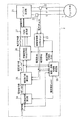•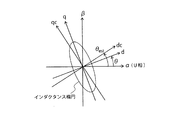•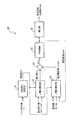•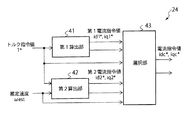•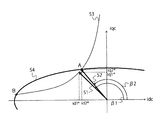•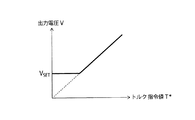•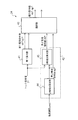••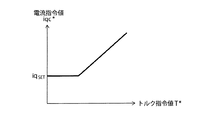•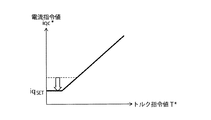•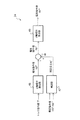•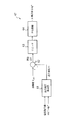••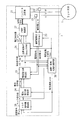•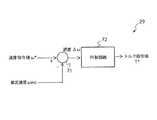•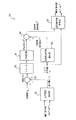## Classifications

• HELECTRICITY
• H02GENERATION; CONVERSION OR DISTRIBUTION OF ELECTRIC POWER
• H02PCONTROL OR REGULATION OF ELECTRIC MOTORS, ELECTRIC GENERATORS OR DYNAMO-ELECTRIC CONVERTERS; CONTROLLING TRANSFORMERS, REACTORS OR CHOKE COILS
• H02P6/00Arrangements for controlling synchronous motors or other dynamo-electric motors using electronic commutation dependent on the rotor position; Electronic commutators therefor
• H02P6/14Electronic commutators
• H02P6/16Circuit arrangements for detecting position
• H02P6/18Circuit arrangements for detecting position without separate position detecting elements
• H02P6/182Circuit arrangements for detecting position without separate position detecting elements using back-emf in windings
• HELECTRICITY
• H02GENERATION; CONVERSION OR DISTRIBUTION OF ELECTRIC POWER
• H02PCONTROL OR REGULATION OF ELECTRIC MOTORS, ELECTRIC GENERATORS OR DYNAMO-ELECTRIC CONVERTERS; CONTROLLING TRANSFORMERS, REACTORS OR CHOKE COILS
• H02P21/00Arrangements or methods for the control of electric machines by vector control, e.g. by control of field orientation
• H02P21/22Current control, e.g. using a current control loop
• HELECTRICITY
• H02GENERATION; CONVERSION OR DISTRIBUTION OF ELECTRIC POWER
• H02PCONTROL OR REGULATION OF ELECTRIC MOTORS, ELECTRIC GENERATORS OR DYNAMO-ELECTRIC CONVERTERS; CONTROLLING TRANSFORMERS, REACTORS OR CHOKE COILS
• H02P21/00Arrangements or methods for the control of electric machines by vector control, e.g. by control of field orientation
• H02P21/24Vector control not involving the use of rotor position or rotor speed sensors
• HELECTRICITY
• H02GENERATION; CONVERSION OR DISTRIBUTION OF ELECTRIC POWER
• H02PCONTROL OR REGULATION OF ELECTRIC MOTORS, ELECTRIC GENERATORS OR DYNAMO-ELECTRIC CONVERTERS; CONTROLLING TRANSFORMERS, REACTORS OR CHOKE COILS
• H02P6/00Arrangements for controlling synchronous motors or other dynamo-electric motors using electronic commutation dependent on the rotor position; Electronic commutators therefor
• H02P6/14Electronic commutators
• H02P6/16Circuit arrangements for detecting position
• H02P6/18Circuit arrangements for detecting position without separate position detecting elements
• HELECTRICITY
• H02GENERATION; CONVERSION OR DISTRIBUTION OF ELECTRIC POWER
• H02PCONTROL OR REGULATION OF ELECTRIC MOTORS, ELECTRIC GENERATORS OR DYNAMO-ELECTRIC CONVERTERS; CONTROLLING TRANSFORMERS, REACTORS OR CHOKE COILS
• H02P25/00Arrangements or methods for the control of AC motors characterised by the kind of AC motor or by structural details
• H02P25/02Arrangements or methods for the control of AC motors characterised by the kind of AC motor or by structural details characterised by the kind of motor
• H02P25/08Reluctance motors

## Description

Embodiments described herein relate generally to an inverter control device and a motor drive system.

Conventionally, in a rotational phase angle sensorless control device of a permanent magnet synchronous motor (PMSM) or a synchronous reluctance motor (SynRM), a rotational phase angle estimation method using an induced voltage is used in a high speed range. However, SynRM and PMSM with a small magnet magnetic flux have a problem that the estimation accuracy of the rotational phase angle is deteriorated because the induced voltage is small in a low load state even in a high speed region.

JP 2003-250293 A JP 2006-74902 A

Provided are an inverter control device and a motor drive system capable of accurately estimating a rotational phase angle of a motor in a high speed and low load state.

An inverter control device according to an embodiment includes an inverter main circuit, a current detector, a current command value calculation unit, a voltage command value calculation unit, and an estimation unit. The inverter main circuit can be electrically connected to a predetermined rotational drive target. The current detector detects a current value output from the inverter main circuit. The current command value calculation unit calculates a current command value at which the output voltage output from the inverter main circuit is equal to or higher than a predetermined target value. The voltage command value calculation unit calculates a voltage command value at which the current value is equal to the current command value. The estimation unit calculates an estimated rotation phase angle of the rotation drive target based on the voltage command value and the current value.

The figure which shows the structure of the motor drive system which concerns on 1st Embodiment. The figure explaining a three-phase fixed coordinate system and a dcqc axis | shaft rotation coordinate system. The figure which shows the structure of the speed and rotational phase angle estimation part of FIG. The figure which shows the structure of the electric current command value calculation part of FIG. The figure explaining the calculation method of an electric current command value. The figure which shows the relationship between torque command value T * of the control apparatus of FIG. 1, and an output voltage. The figure which shows the structure of the modification 1 of an electric current command value calculation part. The figure which shows the structure of the modification 2 of an electric current command value calculation part. The figure explaining the calculation method of the electric current command value in the modification 2. FIG. The figure explaining the calculation method of the electric current command value in the modification 2. FIG. The figure which shows the structure of the modification 3 of an electric current command value calculation part. The figure which shows the structure of a correction | amendment part. The figure which shows the structure of the modification 4 of an electric current command value calculation part. The figure which shows the structure of the motor drive system which concerns on 2nd Embodiment. The figure which shows the structure of the torque command value calculation part of FIG. The figure which shows the structure of the electric current command value calculation part of FIG.

Embodiments of the present invention will be described below with reference to the drawings.

(First embodiment)
A motor drive system (hereinafter referred to as “system”) according to the first embodiment will be described with reference to FIGS. FIG. 1 is a diagram illustrating a configuration of a system according to the present embodiment. As shown in FIG. 1, the system according to the present embodiment includes a motor 1 and an inverter control device 2.

The motor 1 is a rotational drive target of the control device 2 and is connected to the control device 2. Hereinafter, a case where the motor 1 is a synchronous reluctance motor (hereinafter referred to as “SynRM1”) will be described as an example. The SynRM 1 includes a stator and a rotor. The stator has three excitation phases (U phase, V phase, and W phase). The stator generates a magnetic field by a three-phase alternating current flowing in each excitation phase. The rotor does not have a permanent magnet. The rotor rotates due to magnetic interaction with the magnetic field generated by the stator.

The inverter control device 2 (hereinafter referred to as “control device 2”) controls the rotational phase angle of the SynRM 1 without a sensor. As shown in FIG. 1, the control device 2 according to the present embodiment includes an inverter 21, a current detector 22, a coordinate conversion unit 23, a current command value calculation unit 24, a voltage command value calculation unit 25, and a coordinate A conversion unit 26, a PWM modulation unit 27, and a speed / rotation phase angle estimation unit 28 are provided.

The inverter 21 is a circuit including a switching element (transistor). The inverter 21 converts electric power from a power source (not shown) into alternating current by switching ON / OFF of the switching element, and supplies the alternating current to the SynRM 1. The inverter 21 receives a control signal for controlling ON / OFF of each switching element from the PWM modulator 22.

The current detector 22 detects a two-phase or three-phase current value of the three-phase alternating current flowing through the stator of the SynRM 1. FIG. 1 shows a configuration for detecting current values iu and iw of two phases (U phase and W phase).

The coordinate conversion unit 23 converts the current values iu and iw detected by the current detector 22 from the three-phase fixed coordinate system to the dcqc axis rotation coordinate system. Here, the three-phase fixed coordinate system and the dcqc axis rotation coordinate system will be described with reference to FIG.

As shown in FIG. 2, the three-phase fixed coordinate system is a fixed coordinate system composed of an α axis and a β axis. In FIG. 2, the α axis is set in the U-phase direction, and the β axis is set in a direction perpendicular to the α axis. The current values iu and iw detected by the current detector 22 are expressed on such three-phase fixed coordinates.

On the other hand, the dcqc axis rotation coordinate system is a rotation coordinate system including a dc axis and a qc axis. The dc axis is set in the direction estimated by the control device 2 as the d-axis direction (the direction in which the rotor inductance is minimum), and the qc axis is the q-axis direction in the q-axis direction (the direction in which the rotor inductance is maximum). Is set in the estimated direction. The inductance ellipse in FIG. 2 indicates the inductance of the rotor.

As shown in FIG. 2, the dcqc axis and the dq axis do not always coincide with each other. The actual rotational phase angle θ of the rotor is represented by an angle from the α axis to the d axis. Further, the estimated rotational phase angle θest of the rotor estimated by the control device 2 is represented by an angle from the α axis to the dc axis. The closer the angle between the rotational phase angle θ and the estimated rotational phase angle θest, the higher the estimation accuracy of the rotational phase angle.

The coordinate conversion unit 23 can convert the three-phase fixed coordinate system into the dcqc axis rotation coordinate system by using the estimated rotation phase angle θest output from the speed rotation phase angle estimation unit 28. Hereinafter, the current values iu and iw coordinate-converted by the coordinate conversion unit 23 are referred to as current values idc and iqc. The current value idc is a dc axis component of the current flowing through the stator, and the current value iqc is a qc axis component of the current flowing through the stator.

The current command value calculation unit 24 calculates current command values idc * and iqc * based on the torque command value T * and the estimated speed ωest. The torque command value T * is a torque value generated in the rotor. In the present embodiment, it is assumed that the torque command value T * is input from an external device. The estimated speed ωest is the angular speed of the rotor estimated by the control device 2. The current command value idc * is a dc axis component of a current that flows through SynRM1. The current command value iqc * is the qc-axis component of the current that flows through SynRM1. Details of the current command value calculation unit 24 will be described later.

The voltage command value calculation unit 25 (current control unit) calculates voltage command values vdc * and vqc * at which the current values idc and iqc of SynRM1 are equal to the current command values idc * and iqc * . The voltage command value vdc * is a dc axis component of the voltage applied to the stator of SynRM1. The voltage command value vqc * is a qc-axis component of the voltage applied to the stator of SynRM1.

The coordinate conversion unit 26 converts the voltage command values vdc * and vqc * output from the voltage command calculation unit 25 from a dcqc axis rotation coordinate system to a three-phase fixed coordinate system. Similar to the coordinate conversion unit 23, the coordinate conversion unit 26 can convert the dcqc axis rotation coordinate system into a three-phase fixed coordinate system by using the estimated rotation phase angle θest. Hereinafter, the voltage command values vdc * and vqc * coordinate-converted by the coordinate conversion unit 26 are referred to as voltage command values vu * , vv * and vw * . The voltage command value vu * is a voltage applied to the U phase of the stator, the voltage command value vv * is a voltage applied to the V phase of the stator, and the voltage command value vw * is a W phase of the stator. Is the voltage applied to.

The PWM modulation unit 27 modulates the voltage command values vu * , vv * , vw * by PWM (Pulse-Width Modulation) using a triangular wave, and outputs a binary value corresponding to ON or OFF of each switching element of the inverter 21. Generate a control signal. The PWM modulation unit 27 inputs the generated control signal to the inverter 21.

The speed / rotation phase angle estimator 28 (hereinafter referred to as “estimator 28”) determines the rotor of the SynRM 1 based on the torque command value T * , the voltage command values vdc * and vqc * , and the current values idc and iqc. The speed ω and the rotational phase angle θ are estimated, and the estimated speed ωest and the estimated rotational phase angle θest are calculated.

FIG. 3 is a diagram illustrating a configuration of the estimation unit 28. As shown in FIG. 3, the estimation unit 28 includes a phase difference δ setting unit 31, a γ voltage calculation unit 32, a γ voltage estimation unit 33, a subtractor 34, a PI controller 35, an integrator 36, Is provided.

The phase difference δ setting unit 31 outputs a phase difference δ corresponding to the torque command value T * from among a plurality of phase differences δ stored in advance. The phase difference δ is a phase difference value or range in which the voltage change is the largest due to the influence of the error Δθ between the rotational phase angle θ and the estimated rotational phase angle θest. The plurality of phase differences δ are calculated in advance analytically or experimentally for each torque value and stored in the phase difference δ setting unit 31.

The γ voltage calculation unit 32 calculates the voltage value vγ of the γ voltage based on the voltage command values vdc * and vqc * and the phase difference δ set (output) by the phase difference δ setting unit 31. The γ voltage is a feature amount that changes according to the error Δθ. The voltage value vγ is calculated by the following equation, for example.

The voltage value vγ calculated by the γ voltage calculation unit 32 is input to the subtractor 34.

The γ voltage estimation unit 33 calculates an estimated voltage value vγest of the γ voltage based on the voltage values vdcest and vqcest and the phase difference δ set (output) by the phase difference δ setting unit 31.

First, the γ voltage estimation unit 33 calculates voltage values vdcest and vqcest based on the current values idc and iqc and the estimated speed ωest. The voltage value vdcest is an estimated value of the dc axis component of the voltage applied to the stator of SynRM1. The voltage value vqcest is an estimated value of the qc-axis component of the voltage applied to the stator of SynRM1. The voltage values vdcest and vqcest are calculated by the following equations.

In Equation (2), Rm is the winding resistance of the stator, Ld is the inductance in the d-axis direction, Lq is the inductance in the q-axis direction, and p is the differential operator (d / dt). The γ voltage estimation unit 33 stores these values in advance.

Next, the γ voltage estimation unit 33 calculates an estimated voltage value vγest of the γ voltage based on the voltage values vdcest, vqcest and the phase difference δ. The estimated voltage value vγest is calculated by the following equation, for example.

The estimated voltage value vγest calculated by the γ voltage estimation unit 33 is input to the subtractor 34.

The subtracter 34 subtracts the voltage value vγ from the estimated voltage value vγest to calculate an error Δvγ of the γ voltage. Since the γ voltage changes according to the error Δθ, the error Δvγ is proportional to the error Δθ. The error Δvγ calculated by the subtractor 34 is input to the PI controller 35.

Note that the linearity of the error Δvγ with respect to the error Δθ can be improved by calculating the γ voltage using the equation (1). That is, the range of the error Δθ in which the error Δvγ is proportional to the error Δθ can be expanded.

The PI controller 35 performs the PI control so that the error Δvγ becomes 0, thereby estimating the rotor speed ω and calculating the estimated speed ωest. The estimated speed ωest calculated by the PI controller 35 is sequentially fed back to the γ voltage estimator 33 and input to the integrator 36.

The integrator 36 integrates the estimated speed ωest calculated by the PI controller 35 to calculate the estimated rotational phase angle θest.

With the configuration as described above, the estimation unit 28 can calculate the estimated speed ωest and the estimated rotational phase angle θest. The estimated speed ωest calculated by the estimation unit 28 is input to the current command value calculation unit 24. The estimated rotational phase angle θest is input to the coordinate conversion units 23 and 26 and used for coordinate conversion.

Note that the estimation method of the speed ω and the rotation phase angle θ by the estimation unit 28 is not limited to this, and can be arbitrarily selected from known estimation methods. For example, the estimation unit 28 may estimate the rotational phase angle θ by another method using a voltage generated by the flux linkage, or may estimate the rotation phase angle θ by using the flux linkage itself. Then, the rotational phase angle θ may be estimated by performing PI control so that the deviation of the q-axis component of the current value becomes zero.

Here, the current command value calculation unit 24 will be described in detail. The current command value calculation unit 24 according to the present embodiment calculates the current command value so that the output voltage of the SynRM 1 is equal to or higher than a predetermined target value V SET . The target value V SET is a voltage value obtained experimentally or analytically in advance as an output voltage capable of accurately estimating the speed ω and the rotational phase angle θ.

Here, FIG. 4 is a diagram illustrating a configuration of the current command value calculation unit 24. As shown in FIG. 4, the current command value calculation unit 24 includes a first calculation unit 41, a second calculation unit 42, and a selection unit 43.

The first calculator 41 generates first current command values id1 * and iq1 * based on the torque command value T * . The first current command value id1 * is a dc-axis component of a current that flows through SynRM1. The first current command value iq1 * is a qc-axis component of a current that flows through SynRM1. The first calculator 41 calculates the first current command values id1 * and iq1 * so that the torque of SynRM1 becomes the torque command value T * .

FIG. 5 is a diagram for explaining a method of calculating a current command value. In FIG. 5, the horizontal axis is idc, the vertical axis is iqc, the arrow 51 is the first current vector, the arrow 52 is the second current vector, the curve 53 is the equal torque curve of the torque command value T * , and the curve 62 is the target value V. It is an isovoltage ellipse of SET .

The first current vector 51 is a vector corresponding to the first current command values id1 * and iq1 * . In the above description, the first current command value is represented by two values (id1 * , iq1 * ). However, the current magnitude (id1 * 2 + iq1 * 2 ) 1/2 , the current phase angle β1, It is also possible to express by. These representations can be converted to each other. Selecting an arbitrary point from the plane of FIG. 5 corresponds to calculating (selecting) the first current command value.

As shown in FIG. 5, the first calculator 41 selects an arbitrary point on the equal torque curve 53 of the torque command value T * as the first current command value. The first calculator 41 can select the first current command value from the equal torque curve 53 by an arbitrary method according to the control desired to be realized by the control device 2.

For example, the first calculation unit 41 selects the first current command value so that the magnitude of the current flowing through the stator (the magnitude of the first current vector) is minimized. In this case, the first calculation unit 41 may select the first current command value at which the current phase angle β1 is 135 degrees ignoring the magnetic saturation, or the current phase angle β1 in consideration of the magnetic saturation. May be selected as the first current command value at an angle more than 135 degrees. The first calculation unit 41 may select the first current command value so that the efficiency and power factor of the SynRM 1 are maximized.

The first calculation unit 41 may select the first current command value as described above with reference to a table in which the first current command value for each torque value is stored, or may be obtained by calculation. The first current command value calculated by the first calculation unit 41 is input to the selection unit 43.

The second calculator 42 calculates the second current command values id2 * and iq2 * based on the torque command value T * and the estimated speed ωest. The second current command value id2 * is a dc-axis component of a current that flows through SynRM1. The current command value iq2 * is the qc-axis component of the current that flows through SynRM1. The second calculation unit 42, as the torque of the SynRM1 is the torque command value T *, and, as the output voltage of SynRM1 becomes the target value V SET, the second current command value id2 *, calculates the iq2 * .

The second current vector 52 in FIG. 5 is a vector corresponding to the second current command values id2 * and iq2 * . In the above description, the second current command value is represented by two values (id2 * , iq2 * ), but the current magnitude (id2 * 2 + iq2 * 2 ) 1/2 , the current phase angle β2, It is also possible to express by. These representations can be converted to each other. Selecting an arbitrary point from the plane of FIG. 5 corresponds to calculating (selecting) the second current command value.

As illustrated in FIG. 5, the second calculation unit 42 uses any one of intersections of the equal torque curve 53 of the torque command value T * and the equal voltage ellipse 54 of the target value V SET as the second current command value. Select one. When there are two intersections A and B, the second calculation unit 42 preferably selects the intersection A as shown in FIG.

The intersection A is an intersection on the iqc axis side (q axis side) of the two intersections. In general, the equal voltage ellipse 54 is wide in the d-axis direction and narrow in the q-axis direction. Therefore, when the intersection point A on the q-axis side is selected, the current flowing through the stator is larger than when the intersection point B on the d-axis side is selected. The magnitude (the magnitude of the second current vector) becomes small. For this reason, when the 2nd calculation part 42 selects the intersection A, the power of the control apparatus 2 can be saved. In the following, it is assumed that the second current command value is the intersection A.

The second calculation unit 42 may select the second current command value as described above with reference to a table in which the second current command value for each torque value is stored, or solve the following equation: You may ask for it.

The second current command value calculated by the second calculation unit 42 is input to the selection unit 43.

The selector 43 outputs the first current command value id1 * , iq1 * or the second current command value id2 * , iq2 * as the current command value idc * , iqc * . First, the selection unit 43, the first current command value id1 *, iq1 * is output voltages V 1 corresponding to determines whether less than the target value V SET. The output voltage V 1, is that of SynRM1 the output voltage when the output of the first current command value as a current command value. The determination method will be described later.

Next, the selection unit 43 selects one of the first current command values id1 * and iq1 * and the second current command values id2 * and iq2 * according to the determination result, and outputs the selected current command values idc * and iqc *. To do.

If the output voltage V 1 is lower than the target value V SET (V 1 <V SET ), the selection unit 43, the second current command value id2 *, iq2 * of the current command value idc *, selected as iqc *. The case where the output voltage V 1 is lower than the target value V SET is a case where the first current command value is included in the equal voltage ellipse 54 as shown in FIG.

At this time, the second current command value is closer to the iqc axis (q axis) than the first current command value. That is, the current phase angle β2 of the second current command value is on the iqc axis (q axis) side from the current phase angle β1 of the first current command value. The q-axis component iq2 * of the second current command value is larger than the q-axis component iq1 * of the first current command value, and the d-axis component id2 * of the second current command value is the d-axis of the first current command value. Less than component id1 * .

On the other hand, when the output voltage V 1 is equal to or higher than the target value V SET (V 1 ≧ V SET ), the selection unit 43 selects the first current command values id1 * and iq1 * as the current command values idc * and iqc * . The case where the output voltage V 1 is equal to or higher than the target value V SET is a case where the second current command value is on the equal voltage ellipse 54 or outside the equal voltage ellipse 54.

At this time, the first current command value is closer to the iqc axis (q axis) than the second current command value. That is, the current phase angle β1 of the first current command value is on the iqc axis (q axis) side from the current phase angle β2 of the second current command value. Further, q-axis component of the first current command value iq1 * is, q-axis component iq2 * greater second current command value, d-axis component of the first current command value id1 * is, d-axis of the second current command value Less than component id2 * .

The selection unit 43 outputs the selected first current command value or second current command value as a current command value. The current command value output by the selection unit 43 is input to the voltage command calculation unit 25.

Next, a method for determining whether or not the output voltage V 1 is lower than the target value V SET by the selection unit 43 will be described.

Selection unit 43, for example, acquires the output voltage V 1, by comparing the target value V SET, a determination. The selection unit 43 may select the output voltage V 1 with reference to a table in which the output voltage for each first current command value is stored, or may be calculated by the following equation.

Further, the selection unit 43 may perform the determination by comparing the current phase angle β1 of the first current command value with the current phase angle β2 of the second current command value. Selection unit 43, when the current phase angle β2 of the q-axis side from the current phase angle .beta.1, determines the output voltage V 1 is lower than the target value V SET.

Further, the selection unit 43, a q-axis component iq1 * of the first current command value, the q-axis component iq2 * of the second current command value, by comparing may perform determination. Selection unit 43, when the q-axis component iq1 * is the q-axis component iq2 * smaller, determines the output voltage V 1 is lower than the target value V SET.

Furthermore, the selection unit 43 may perform the determination by comparing the d-axis component id1 * of the first current command value with the d-axis component id2 * of the second current command value. Selection unit 43 determines a d-axis component id1 * if d-axis component id2 * is greater than the output voltage V 1 is lower than the target value V SET.

The current command value calculation unit 24 calculates the current command values idc * and iqc * in this way, so that the output voltage of the SynRM1 is set as a target for an arbitrary torque command value T * as shown by a solid line in FIG. It can be greater than or equal to the value V SET .

In the conventional control device, even when SynRM1 operates in the high speed region, the output voltage (induced voltage) of SynRM1 is small in the low load state where the torque command value T * is small, as shown by the broken line in FIG. . For this reason, it is difficult to accurately estimate the speed ω and the rotational phase angle θ using the induced voltage.

However, since the control device 2 according to the present embodiment can set the output voltage to be equal to or higher than the target value V SET even in the case of a low load, based on the output voltage of SynRM1, the speed ω and rotation of the SynRM1 The phase angle θ can be estimated with high accuracy.

In the above description, the case where the control device 2 controls the operation of the SynRM 1 has been described. However, the control device 2 can also be used as a PMSM control device with a small magnet magnetic flux. PMSM having a small magnet magnetic flux has a low induced voltage at low load, similar to SynRM1. By applying the control device 2 according to the present embodiment to such a PMSM, it is possible to improve the accuracy of estimation of the PMSM speed ω and the rotational phase angle θ when the load is low.

(Modification 1 of the first embodiment)
Modification 1 of the current command value calculation unit 24 according to the present embodiment will be described with reference to FIG. FIG. 7 is a diagram illustrating a configuration of the first modification of the current command value calculation unit 24. As shown in FIG. 7, the second calculation unit 43 includes a q-axis component calculation unit 44 and a d-axis component calculation unit 45. Other configurations of the current command value calculation unit 24 are the same as those in FIG.

The q-axis component calculation unit 44 calculates the q-axis component iq2 * of the second current command value based on the estimated speed ωest. Specifically, the q-axis component calculation unit 44, the current value iq2 * to calculate the q-axis component iq2 * to be inversely proportional with the estimated speed .omega.est. The q-axis component iq2 * is calculated by the following equation, for example.

The d-axis component calculation unit 45 calculates the d-axis component id2 * of the second current command value based on the torque command value T * and the q-axis component iq2 * . The d-axis component id2 * is calculated by the following equation, for example.

With such a configuration, the control device 2 can set the output voltage at a low load to be equal to or higher than the target value V SET . In addition, current loss in the high speed region can be suppressed.

(Modification 2 of the first embodiment)
Modification 2 of the current command value calculation unit 24 according to the present embodiment will be described with reference to FIGS. FIG. 8 is a diagram illustrating a configuration of a second modification of the current command value calculation unit 24. As shown in FIG. 8, the current command value calculation unit 24 calculates current command values iqc * and idc * based on the torque command value T * and the estimated speed ωest.

In this modification, the current command value calculation unit 24 calculates the q-axis component iqc * such that the q-axis component iqc * of the current command value is equal to or greater than a predetermined target value Iq SET , as shown in FIG. . Further, as shown in FIG. 10, the current command value calculation unit 24 generates the q-axis component iqc * so that the target value Iq SET decreases as the estimated speed ωest increases.

With such a configuration, the motor output voltage at the time of low load can be set to the target value V SET or more. In addition, current loss in the high speed region can be suppressed.

(Modification 3 of the first embodiment)
Modification 3 of the current command value calculation unit 24 according to the present embodiment will be described with reference to FIGS. 11 and 12. The current command value calculation unit 24 described above calculates a current command value at which the output voltage is equal to or higher than the target value V SET by selecting one of the two current command values. On the other hand, in this modification, the current command value at which the output voltage is equal to or higher than the target value V SET is calculated by correcting one current command value.

FIG. 11 is a diagram illustrating a configuration of a third modification of the current command value calculation unit 24. As shown in FIG. 11, the current command value calculation unit 24 includes a q-axis component calculation unit 46, a correction unit 47, an adder 48, and a d-axis component calculation unit 49.

The q-axis component calculation unit 46 calculates a current command value iq3 * based on the torque command value T * . The current command value iq3 * is a value before correction of the q-axis component of the current command value. The current command value calculation unit 24 in the present modification calculates the q-axis component of the current command value by correcting the current command value iq3 * . The current command value iq3 * is calculated by an arbitrary method according to the control to be realized by the control device so that the torque of the SynRM1 becomes the torque command value T * .

For example, the q-axis component calculation unit 46 calculates the current command value iq3 * so that the magnitude of the current flowing through the stator is minimized. In this case, the q-axis component calculation unit 46 may calculate the current command value iq3 * at which the current phase angle β is 135 degrees, ignoring the magnetic saturation, or the current phase angle in consideration of the magnetic saturation. A current command value iq3 * at which β is an angle advanced from 135 degrees may be calculated. In addition, the q-axis component calculation unit 46 may calculate the current command value iq3 * so that the efficiency and power factor of SynRM1 are maximized.

The current command value iq3 * calculated by the q-axis component calculation unit 46 is input to the adder 48.

The correction unit 47 receives the voltage command values vdc * and vqc * from the voltage command value calculation unit 25. Correction unit 47 calculates correction value Δiq * based on voltage command values vdc * and vqc * . Correction value? Iq * corrects the current command value IQ3 *, a current command value for calculating a q-axis component of the current command value iqc *. Details of the correction unit 47 will be described later. The correction value Δiq * calculated by the correction unit 47 is input to the adder 48.

The adder 48 adds the correction value? Iq * to the current command value IQ3 *, calculates the current command value iqc *. The current command value iqc * calculated by the adder 48 is input to the d-axis component calculation unit 49.

The d-axis component calculation unit 49 calculates the d-axis component idc * of the current command value based on the torque command value T * and the current command value iqc * so that the torque of the SynRM1 becomes the torque command value T *. calculate. The d-axis component idc * is calculated by the following equation, for example.

Here, the correction unit 47 will be described with reference to FIG. FIG. 12 is a diagram illustrating a configuration of the correction unit 47. As illustrated in FIG. 12, the correction unit 47 includes an output voltage calculation unit 61, a subtracter 62, a limiter 63, and a PI controller 64.

The output voltage calculation unit 61 receives the voltage command values vdc * and vqc * calculated by the voltage command value calculation unit 25. Output voltage calculation unit 61, voltage command value vdc *, based on vqc *, calculates the output voltage V 1 of the SynRM1. Output voltages V 1, for example, is calculated by the following equation.

The output voltage V 1 calculated by the output voltage calculation unit 61 is input to the subtractor 62.

The subtractor 62 subtracts the output voltage V 1 from the output voltage target value V SET to calculate an output voltage error ΔV. The error ΔV calculated by the subtractor 62 is input to the limiter 63.

The limiter 63 limits the error ΔV to 0 or more. That is, only an error ΔV of 0 or more is output. The error ΔV output from the limiter 63 is input to the PI controller 64.

The PI controller 64 performs PI control based on the error ΔV limited to 0 or more, and calculates a correction value Δiq * at which the output voltage V 1 of the SynRM 1 is equal to or higher than the target value V SET . The correction value Δiq * calculated by the PI controller 64 is input to the adder 48.

With such a configuration, when the output voltage V 1 corresponding to the current command value is lower than the target value V SET , that is, when ΔV is equal to or greater than 0, the current command is set so that the output voltage V 1 is equal to or greater than the target value V SET. The correction value Δiq * is added to the value as an offset current. Thus, it is possible to output voltage V 1 of the low load and the target value V SET more.

(Modification 4 of the first embodiment)
Modification 4 of the current command value calculation unit 24 according to the present embodiment will be described with reference to FIG. In the present modification, as in Modification 3, by correcting one current command value, a current command value at which the output voltage is equal to or higher than the target value V SET is calculated. In the third modification, a correction value Δiq * is generated by the correction unit 47 and added to the current command value iq3 * by the adder 48 to generate a current command value iqc * . In contrast, in this modification, the lower limiter 91, by limiting the lower limit of the current command value IQ3 *, generates a current command value iqc *.

Lower limiter 91, as shown in FIG. 9, as iqc * becomes equal to or higher than iq SET, to IQ3 * correction (limit). For example, iq SET is calculated from the minimum speed ω MIN used in the present embodiment and the output voltage target value V SET by the following equation.

With such a configuration, the output voltage V 1 can be made equal to or higher than the target value V SET even when the load is low. In the present modification, the output voltage V 1 can be made equal to or higher than the target value V SET with a simple configuration in which only the lower limiter 91 is added to the first embodiment and Modifications 1 to 3.

(Second Embodiment)
Next, the control apparatus 2 which concerns on 2nd Embodiment is demonstrated with reference to FIGS. FIG. 14 is a diagram illustrating a configuration of a system according to the present embodiment. As shown in FIG. 14, the control device 2 according to the present embodiment further includes a torque command value calculation unit 29. Other configurations are the same as those of the first embodiment.

The torque command value calculation unit (speed controller) 29 calculates a torque command value T * based on the speed command value ω * and the estimated speed ωest. The speed command value ω * is an angular speed for rotating the rotor of SynRM1. In the present embodiment, the torque command value T * calculated by the torque command value calculation unit 29 is input to the current command value calculation unit 24. In the present embodiment, the speed command value ω * is input from an external device.

FIG. 15 is a diagram illustrating a configuration of the torque command value calculation unit 29. As shown in FIG. 15, the torque command value calculation unit 29 includes a subtracter 71 and a PI controller 72.

The subtracter 71 receives the speed command value ω * and the estimated speed ωest. The subtracter 71 subtracts the estimated speed ωest from the speed command value ω * to calculate an angular speed error Δω of the rotor. The error Δω calculated by the subtracter 71 is input to the PI controller 72.

The PI controller 72 performs PI control based on the error Δω, and calculates a torque command value T * at which the error Δω becomes zero. The torque command value T * calculated by the PI controller 72 is input to the current command value calculation unit 24.

As described above, the current command value calculation unit 24 may have the configuration described in the first embodiment, but may have another configuration. FIG. 16 is a diagram illustrating another configuration of the current command value calculation unit 24. The current command value calculation unit 24 calculates current command values idc * and idc * based on the torque command value T * and the phase angle command value β * .

As shown in FIG. 16, the current command value calculation unit 24 includes an output voltage calculation unit 81, a subtracter 82, a limiter 83, a PI controller 84, a phase angle command value calculation unit 85, and a subtractor 86. And a current command value acquisition unit 87.

The output voltage calculation unit 81 receives the voltage command values vdc * and vqc * calculated by the voltage command value calculation unit 25. Output voltage calculation unit 81, voltage command value vdc *, based on vqc *, calculates the output voltage V 1 of the SynRM1. The output voltage V 1 calculated by the output voltage calculation unit 81 is input to the subtractor 82.

The subtracter 82 subtracts the output voltage V 1 from the output voltage target value V SET to calculate an output voltage error ΔV. The error ΔV calculated by the subtractor 82 is input to the limiter 83.

The limiter 83 limits the error ΔV to 0 or more. That is, only an error ΔV of 0 or more is output. The error ΔV output from the limiter 83 is input to the PI controller 84.

The PI controller 84 performs PI control based on the error ΔV limited to 0 or more, and calculates a correction value Δβ * at which the output voltage V 1 of the SynRM 1 is equal to or higher than the target value V SET . Correction value [Delta] [beta] * is the phase angle command value to calculate the phase angle command value beta * to correct the phase angle command value .beta.1 *. The correction value Δβ * calculated by the PI controller 84 is input to the subtractor 86.

The phase angle command value calculation unit 85 calculates the phase angle command value β1 * based on the torque command value T * . The phase angle command value β1 * is a value before correction of the phase angle command value β * . The phase angle command value β1 * is calculated by an arbitrary method according to the control desired to be realized by the control device so that the torque of the SynRM1 becomes the torque command value T * .

For example, the phase angle command value calculation unit 85 calculates the phase angle command value β1 * so that the magnitude of the current flowing through the stator is minimized. In this case, the phase angle command value calculation unit 85 may ignore the magnetic saturation and set the phase angle command value β1 * to 135 degrees, or consider the magnetic saturation and set the phase angle command value β1 * from 135 degrees. It may be an advanced angle. Further, the phase angle command value calculation unit 85 may calculate the phase angle command value β1 * so that the efficiency and the power factor of SynRM1 are maximized.

The phase angle command value β1 * calculated by the phase angle command value calculation unit 85 is input to the subtractor 86.

The subtracter 86 subtracts the correction value Δβ * from the phase angle command value β1 * to calculate the phase angle command value β * . The phase angle command value β * calculated by the subtractor 86 is input to the current command value acquisition unit 87.

The current command value acquisition unit 87 calculates current command values idc * and iqc * based on the torque command value T * and the phase angle command value β * . Specifically, the current command value acquisition unit 87 may acquire the coordinates of the point where the current phase angle becomes the phase angle command value β * on the equal torque curve of the torque command value T * . The current command values idc * and iqc * acquired by the current command value acquisition unit 87 are input to the voltage command value calculation unit 25.

As described above, the current command value calculation unit 24 in FIG. 16 corrects the current phase angle so that the output voltage V 1 is equal to or higher than the target value V SET . Specifically, the current phase angle is corrected in the delay direction so that the current command value moves to the q-axis side. Thus, it is possible to output voltage V 1 of the low load and the target value V SET more.

In addition, the control device 2 according to the present embodiment has lower required accuracy of torque control than the first embodiment. This is because the control device 2 according to the present embodiment performs control so that the speed ω of the SynRM 1 becomes the speed command value ω * .

For this reason, the current command value calculation unit 24 can calculate the current command value without using a table (such as a table in which the output voltage for each current command value is stored) for accurately controlling the torque of the SynRM1. .

Note that the present invention is not limited to the above-described embodiments as they are, and can be embodied by modifying the components without departing from the scope of the invention in the implementation stage. Moreover, various inventions can be formed by appropriately combining a plurality of constituent elements disclosed in the above embodiments. Further, for example, a configuration in which some components are deleted from all the components shown in each embodiment is also conceivable. Furthermore, you may combine suitably the component described in different embodiment.

1: synchronous reluctance motor (SynRM), 2: inverter control device, 21: inverter, 22: current detector, 23: coordinate conversion unit, 24: current command value calculation unit, 25: voltage command value calculation unit, 26: Coordinate conversion unit, 27: PWM modulator, 28: Speed / rotation phase angle estimation unit, 29: Torque command value calculation unit, 31: Phase difference δ setting unit, 32: γ voltage calculation unit, 33: γ voltage estimation unit, 34: subtractor, 35: PI controller, 36: integrator, 41: first calculator, 42: second calculator, 43: selector, 44: q-axis component calculator, 45: d-axis component calculator 46: q-axis component calculation unit, 47: correction unit, 48: adder, 49d-axis component calculation unit, 51: first current vector, 52: second current vector, 53: isotorque curve, 54: isovoltage ellipse 61: output voltage calculation unit, 62: subtractor, 63 Limiter, 64: PI controller, 71: Subtractor, 72: PI controller, 81: Output voltage calculator, 82 Subtractor, 83: Limiter, 84: PI controller, 85: Phase angle command value calculator, 86 : Subtractor, 87: Current command value acquisition unit

## Claims (13)

1. An inverter main circuit for driving the rotor of the synchronous reluctance motor ;
A current detector for detecting a current value output from the inverter main circuit;
A current command value calculation unit for calculating a current command value for causing an output voltage generated in a stator of the synchronous reluctance motor to be a predetermined value or more by a linkage magnetic flux generated by a current output from the inverter main circuit;
A voltage command value calculation unit that calculates a voltage command value so that the current value is equal to the current command value;
An estimation unit that calculates an estimated rotational phase angle of a rotor of the synchronous reluctance motor based on the voltage command value and the current value;
An inverter control device comprising:
2. The current command value calculation unit calculates a first current command value based on the torque command value, and calculates a second current command value based on the torque command value and the estimated speed, respectively, and according to the first current command value It is determined whether the output voltage is lower than the predetermined value. When the output voltage is equal to or higher than the predetermined value, the first current command value is output as the current command value. When the output voltage is lower than the predetermined value, the second current command value is The inverter control device according to claim 1, wherein the inverter control device outputs the current command value.
3.   The inverter control device according to claim 2, wherein the output voltage corresponding to the second current command value is the predetermined value.
4. The current command value calculation unit calculates a first current command value based on the torque command value, and calculates a second current command value based on the torque command value and the estimated speed, respectively. 2. The inverter control device according to claim 1, wherein, among the two current command values, the current phase angle closer to the q axis is output as the current command value.
5. The current command value calculation unit calculates a first current command value based on the torque command value, and calculates a second current command value based on the torque command value and the estimated speed, respectively. 2. The inverter control device according to claim 1, wherein, among the two current command values, the one having a larger q-axis component is output as the current command value.
6. The current command value calculation unit performs the determination by comparing a voltage value of the output voltage calculated based on the first current command value with the predetermined value. Inverter control device.
7. The inverter control device according to claim 2, wherein the current command value calculation unit performs the determination by comparing a d-axis component of the first current command value and a d-axis component of the second current command value. .
8. 5. The inverter control device according to claim 4, wherein the current command value calculation unit makes a determination by comparing a current phase angle of the first current command value with a current phase angle of the second current command value.
9. The current command value calculation unit calculates a correction value based on an error between the output voltage and the predetermined value when the output voltage is lower than the predetermined value, and adds the correction value to the current command value. The inverter control device according to Item 1.
10. The inverter control device according to any one of claims 2 to 8, further comprising a torque command value calculation unit that calculates the torque command value based on a speed command value and an estimated speed.
11. The inverter control device according to claim 1, wherein the current command value calculation unit includes a lower limit limiter that limits a lower limit of the current command value.
12. The inverter control device according to claim 11, wherein the lower limiter is set so that the output voltage at a minimum speed becomes the predetermined value.
13. A synchronous reluctance motor,
An inverter main circuit for driving the synchronous reluctance motor;
A current detector for detecting a current value output from the inverter main circuit;
A current command value calculation unit for calculating a current command value for causing an output voltage generated in a stator of the synchronous reluctance motor to be a predetermined value or more by a linkage magnetic flux generated by a current output from the inverter main circuit;
A voltage command value calculation unit that calculates a voltage command value so that the current value is equal to the current command value;
An estimation unit that calculates an estimated rotational phase angle of a rotor of the synchronous reluctance motor based on the voltage command value and the current value;
A motor drive system comprising:
JP2016535264A 2015-01-28 2016-01-26 Inverter control device and motor drive system Active JP6367332B2 (en)

## Priority Applications (3)

Application Number Priority Date Filing Date Title
JP2015014722 2015-01-28
JP2015014722 2015-01-28
PCT/JP2016/052161 WO2016121751A1 (en) 2015-01-28 2016-01-26 Inverter control apparatus and motor driving system

## Publications (2)

Publication Number Publication Date
JPWO2016121751A1 JPWO2016121751A1 (en) 2017-04-27
JP6367332B2 true JP6367332B2 (en) 2018-08-01

# Family

## Family Applications (1)

Application Number Title Priority Date Filing Date
JP2016535264A Active JP6367332B2 (en) 2015-01-28 2016-01-26 Inverter control device and motor drive system

## Country Status (5)

US (1) US10158305B2 (en)
EP (1) EP3252941A4 (en)
JP (1) JP6367332B2 (en)
CN (1) CN107078674B (en)
WO (1) WO2016121751A1 (en)

## Families Citing this family (10)

* Cited by examiner, † Cited by third party
Publication number Priority date Publication date Assignee Title
US9933842B2 (en) 2016-04-15 2018-04-03 Emerson Climate Technologies, Inc. Microcontroller architecture for power factor correction converter
US10284132B2 (en) 2016-04-15 2019-05-07 Emerson Climate Technologies, Inc. Driver for high-frequency switching voltage converters
US10656026B2 (en) 2016-04-15 2020-05-19 Emerson Climate Technologies, Inc. Temperature sensing circuit for transmitting data across isolation barrier
US10305373B2 (en) 2016-04-15 2019-05-28 Emerson Climate Technologies, Inc. Input reference signal generation systems and methods
US20170302200A1 (en) 2016-04-15 2017-10-19 Emerson Climate Technologies, Inc. Buck-converter-based drive circuits for driving motors of compressors and condenser fans
US10277115B2 (en) 2016-04-15 2019-04-30 Emerson Climate Technologies, Inc. Filtering systems and methods for voltage control
TWI668953B (en) * 2016-08-22 2019-08-11 日商東芝股份有限公司 Inverter control device and drive system
TWI654827B (en) * 2016-09-05 2019-03-21 日商東芝股份有限公司 Converter control device and motor driving system
JP2018042324A (en) * 2016-09-05 2018-03-15 東芝インフラシステムズ株式会社 Inverter controller and motor drive system
JP2020031454A (en) * 2016-12-28 2020-02-27 アルプスアルパイン株式会社 Device and method for acquiring information about rotation of dc commutator motor

## Family Cites Families (16)

* Cited by examiner, † Cited by third party
Publication number Priority date Publication date Assignee Title
JP4061517B2 (en) * 1998-07-16 2008-03-19 株式会社安川電機 AC motor variable speed controller
JP3410451B2 (en) * 2000-03-25 2003-05-26 エルジー電子株式会社 Speed controller for synchronous reluctance motor
KR100421373B1 (en) * 2001-06-20 2004-03-06 엘지전자 주식회사 Apparatus for rotary velocity control of synchronous reluctance motor
EP1411629A1 (en) * 2001-07-04 2004-04-21 Kabushiki Kaisha Yaskawa Denki Method and device for controlling currents of synchronous motor
JP3692085B2 (en) 2002-02-21 2005-09-07 株式会社東芝 Motor control method and apparatus
JP4357967B2 (en) * 2002-03-22 2009-11-04 パナソニック株式会社 Control device for synchronous reluctance motor
JP4579627B2 (en) 2004-09-02 2010-11-10 三菱電機株式会社 Rotating machine control device
JP4715158B2 (en) * 2004-10-19 2011-07-06 富士電機システムズ株式会社 Variable speed control device for induction motor
JP5267848B2 (en) * 2008-04-15 2013-08-21 株式会社ジェイテクト Motor control device
JP2010119284A (en) * 2008-10-16 2010-05-27 Panasonic Corp Motor driving device
JP4746667B2 (en) * 2008-11-26 2011-08-10 本田技研工業株式会社 Motor phase current estimation device and motor magnetic pole position estimation device
JP2010136586A (en) * 2008-12-08 2010-06-17 Honda Motor Co Ltd Magnetic pole position estimator for electric motor
WO2010070723A1 (en) * 2008-12-15 2010-06-24 三菱電機株式会社 Power converter for driving motor
JP5971707B2 (en) * 2011-08-29 2016-08-17 株式会社東芝 Sensorless control device and inverter device for synchronous motor
DE112014000512T5 (en) * 2013-03-28 2015-10-15 Aisin Aw Co., Ltd. Device for controlling a rotating electrical machine
JP6193006B2 (en) * 2013-06-20 2017-09-06 株式会社東芝 Electric vehicle control device

## Also Published As

Publication number Publication date
US20170317623A1 (en) 2017-11-02
WO2016121751A1 (en) 2016-08-04
CN107078674A (en) 2017-08-18
EP3252941A1 (en) 2017-12-06
CN107078674B (en) 2019-09-17
EP3252941A4 (en) 2018-10-03
US10158305B2 (en) 2018-12-18
JPWO2016121751A1 (en) 2017-04-27

## Similar Documents

Publication Publication Date Title
US6674262B2 (en) Motor control apparatus and motor control method
US7504797B2 (en) Motor control device
US6690137B2 (en) Sensorless control system for synchronous motor
JP5644820B2 (en) Motor control device
US6771039B2 (en) Motor control apparatus and method
KR101109067B1 (en) Position sensorless control apparatus of permanent magnet motor
EP2892147B1 (en) Electric motor control device
JP4284355B2 (en) High response control device for permanent magnet motor
JP4531751B2 (en) Synchronous machine controller
JP4895703B2 (en) Motor control device
KR100455630B1 (en) Sensorless control method and apparatus of permanent magnet synchronous motor
JP4063166B2 (en) Electric motor control device
US7932692B2 (en) Control system for rotary electric machine with salient structure
JP6014401B2 (en) Electric motor control device
JP4677852B2 (en) Vector controller for permanent magnet synchronous motor
KR100423715B1 (en) Synchronous motor control device and method
JP4655871B2 (en) Field weakening vector control device and module for permanent magnet synchronous motor
JP4881635B2 (en) Vector controller for permanent magnet motor
JP4754417B2 (en) Control device for permanent magnet type rotating electrical machine
US8384322B2 (en) Motor control device and motor drive system
JP5155344B2 (en) Electric motor magnetic pole position estimation device
JP3894286B2 (en) Control device for permanent magnet synchronous motor
JP4641179B2 (en) Synchronous motor control method and control apparatus
JP5761243B2 (en) Motor control device and magnetic pole position estimation method
JP2007282389A (en) Motor control unit

## Legal Events

Date Code Title Description
A131 Notification of reasons for refusal

Free format text: JAPANESE INTERMEDIATE CODE: A131

Effective date: 20170526

A521 Written amendment

Free format text: JAPANESE INTERMEDIATE CODE: A523

Effective date: 20170719

A711 Notification of change in applicant

Free format text: JAPANESE INTERMEDIATE CODE: A712

Effective date: 20170904

A711 Notification of change in applicant

Free format text: JAPANESE INTERMEDIATE CODE: A711

Effective date: 20170905

A131 Notification of reasons for refusal

Free format text: JAPANESE INTERMEDIATE CODE: A131

Effective date: 20171222

A521 Written amendment

Free format text: JAPANESE INTERMEDIATE CODE: A523

Effective date: 20180112

A01 Written decision to grant a patent or to grant a registration (utility model)

Free format text: JAPANESE INTERMEDIATE CODE: A01

Effective date: 20180605

A61 First payment of annual fees (during grant procedure)

Free format text: JAPANESE INTERMEDIATE CODE: A61

Effective date: 20180704